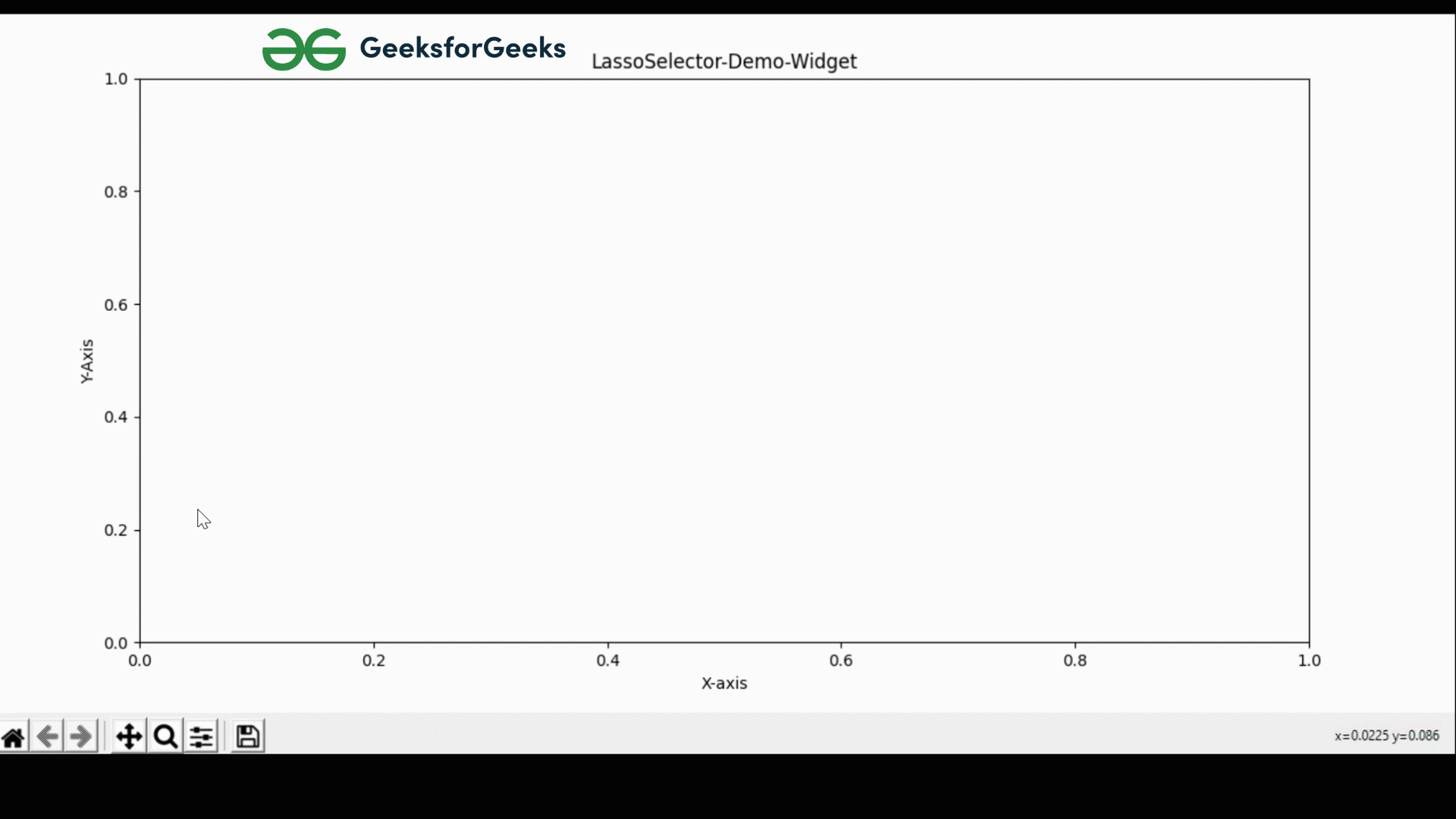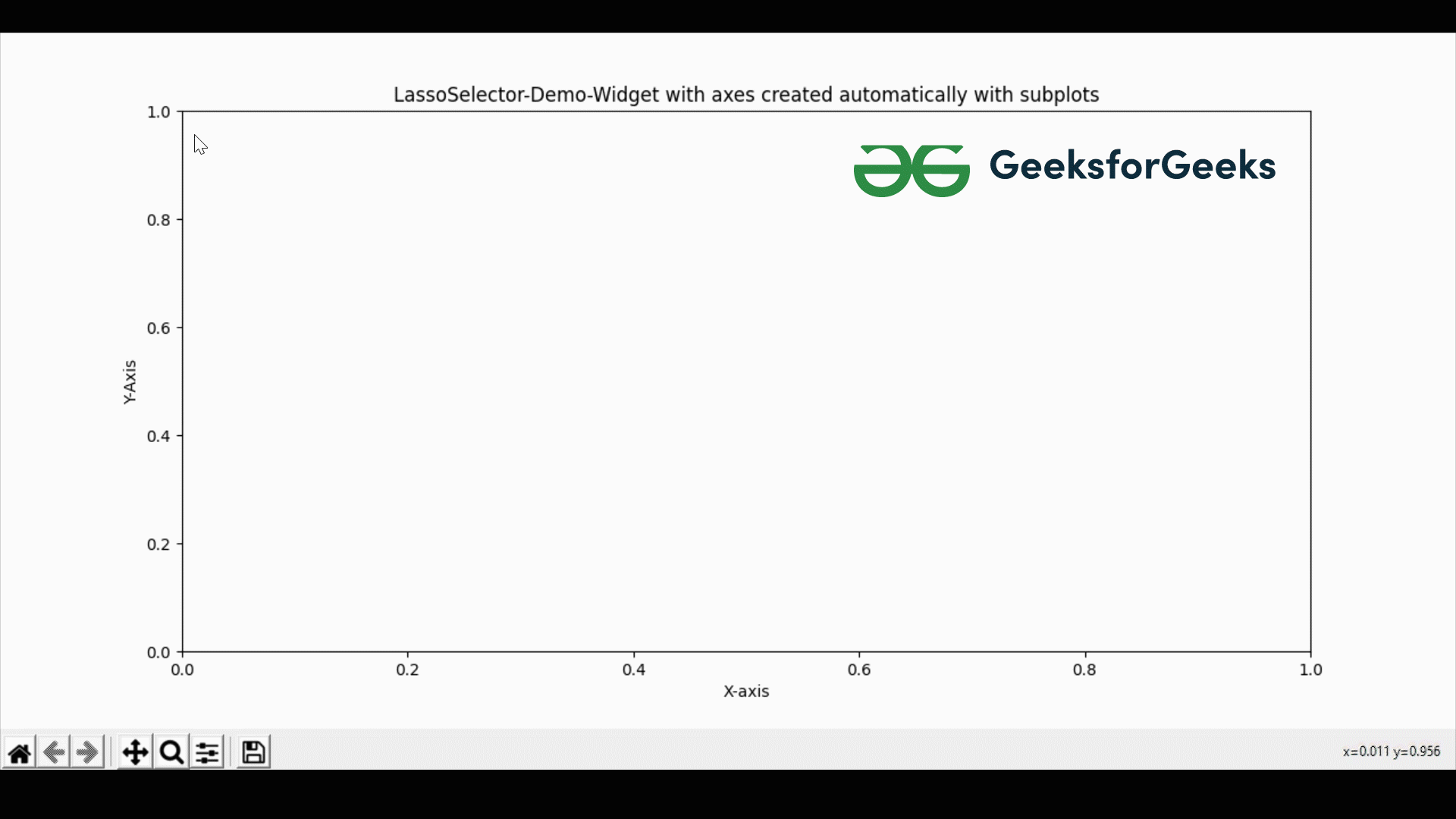Related Articles
Matplotlib – Lasso Selector Widget
• Last Updated : 23 Feb, 2021

Matplotlib provides us with a variety of widgets. In this article, we will be learning about Lasso Selector Widget Demo. A Lasso Selector Widget is a tool that helps us to make a selection curve of arbitrary space.

### Approach #1

We will be adding the axes manually to our plot and then use the lasso-selector-widget tool.

Implementation:

## Python3

 `# importing matplotlib package``import` `matplotlib.pyplot as plt`` ` `# importing LassoSelector from``# Matplotlib.widgets``from` `matplotlib.widgets ``import` `LassoSelector`` ` `# Creating a figure of the plot``fig ``=` `plt.figure()`` ` `# Add set of axes to figure(Manually)``# left, bottom, width, height (ranging in between 0 and 1)``axes ``=` `fig.add_axes([``0.1``, ``0.1``, ``0.8``, ``0.8``])`` ` `# Set the label of X-Axis``axes.set_xlabel(``'X-axis'``)`` ` `# Set the label of Y-Axis``axes.set_ylabel(``'Y-Axis'``)`` ` `# Set the title of the plot``axes.set_title(``'LassoSelector-Demo-Widget'``)`` ` `# OnSelect function:Gets triggered``# as soon as the mouse is pressed``# in the plot``def` `onSelect(geeksforgeeks):``    ``print``(geeksforgeeks)`` ` `# line defines the color, width and opacity``# of the line to be drawn``line ``=` `{``'color'``: ``'green'``, ``        ``'linewidth'``: ``8``, ``'alpha'``: ``1``}`` ` `# Three parameters are passed inside the lasso``# Selector class defining the axis, line``# property and on select function``lsso ``=` `LassoSelector(ax``=``axes, onselect``=``onSelect, ``                     ``lineprops``=``line, button``=``2``)`` ` `# Show the above plot``plt.show()`

Output:Explanation:

In the above code, we are importing matplotlib package to our python project along with the LassoSelector tool from the matplotlib.widgets module. After importing the packages, we are creating a figure(i.e an empty canvas) and adding axes to it manually. Then, we are defining a function onSelect() that gets triggered as soon as the mouse is pressed in the plot. Then, we are creating line that defines the properties of the line, and then comes LassoSelector which helps us to draw inside the plots. Now inside LassoSelector there are four parameters, the first one defines the axes that we have created, the second one defines the onSelect() function, the third parameter is defining the properties of the line(line) and the last parameter defines which click of the mouse will be used to draw the plot(left, right, middle).

### Approach #2

Here, Instead of manually adding axes, we can also do it using plt.subplots which automatically creates axes.

## Python3

 `# importing matplotlib package``import` `matplotlib.pyplot as plt`` ` `# importing LassoSelector from``# Matplotlib.widgets``from` `matplotlib.widgets ``import` `LassoSelector`` ` `# Creating a Subplot in matplotlib``fig, axes ``=` `plt.subplots()`` ` `# Set the label of X-Axis``axes.set_xlabel(``'X-axis'``)`` ` `# Set the label of Y-Axis``axes.set_ylabel(``'Y-Axis'``)`` ` `# Set the title of the plot``axes.set_title(``    ``'LassoSelector-Demo-Widget with axes created automatically with subplots'``)`` ` `# onSelect function gets triggered``# as soon as the mouse is pressed``# in the plot``def` `onSelect(geeksforgeeks):``    ``print``(geeksforgeeks)`` ` `# line defines the color, width and opacity``# of the line to be drawn``line ``=` `{``'color'``: ``'green'``,``        ``'linewidth'``: ``8``, ``'alpha'``: ``1``}`` ` `# Three parameters are passed inside the lasso``# Selector class defining the axis, line``# property and on select function``lsso ``=` `LassoSelector(ax``=``axes, onselect``=``onSelect, ``                     ``lineprops``=``line, button``=``2``)`` ` `# If you want to print x and y while pressing``# and releasing mouse, then use mpl_connect``# and replace pressed and released with event`` ` `# Shows the above plot``plt.show()`

Output:Explanation:

The same series of events are taking place just like our previous approach. The only difference is that here we are automatically creating axes with the help of plt.subplots(). If you go through the terminal, you can see a large number of coordinates which are the points on which we have drawn in the graph.

Attention geek! Strengthen your foundations with the Python Programming Foundation Course and learn the basics.

To begin with, your interview preparations Enhance your Data Structures concepts with the Python DS Course.

My Personal Notes arrow_drop_up